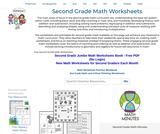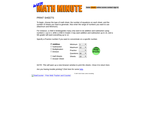# 8 Results

View
Selected filters:
• Math FactsConditional Remix & Share Permitted
CC BY-NC-SA
Rating

The purpose of this lesson is to introduce and activate prior knowledge about addition and subtraction.

Included is a YouTube video to support Grade 3 Blended Learning Math - Unit 3.0: Addition and Subtraction - Introduction

Subject:
Math
Material Type:
Activity/Lab
Homework/Assignment
Lesson
Provider:
Sun West Distance Learning Centre (DLC)
Author:
Sun West School Division
03/14/2019Rating

Challenge first graders with math problems that will help them master first-grade math skills! In first grade, students learn about money, time, measurements, and patterns. Building a solid number sense is important for students in this age range. First graders learn to recognize patterns and sort objects by color, shape, and function. They will also learn to recognize and differentiate between two-dimensional and three-dimensional geometric shapes like squares, triangles, cones, and cylinders. By the end of first grade, students should be able to count to one hundred by ones, twos, fives, and tens. They will also be introduced to the concepts of more and less, and have an opportunity to work with simple graphs.

Now that your child has mastered numbers and counting, it's time to take things to the next level with our first-grade math worksheets! Keep students' math minds sharp with daily first-grade math and critical thinking practice worksheets as well as daily homework and practice pages. Weekly word problems, first-grade algebra and geometry, counting and comparing numbers, and introduction to addition are just some of the engaging, no-prep first-grade math worksheets available on this page.

Subject:
Math
Material Type:
Activity/Lab
Homework/Assignment
Teaching/Learning Strategy
Author:
edHelper
09/14/2020Conditional Remix & Share Permitted
CC BY-NC
Rating

These resources can help you develop a plan for teaching foundational or basic facts in math.

Subject:
Math
Material Type:
Teaching/Learning Strategy
Author:
Melissa Lander
02/07/2020Rating

"Free Printable Math Worksheet Sampler Packs - Need a quick resource for your students to practice their math skills? The sampler packs below are available for free with no login required!"
Jumbo Math Worksheets Pack

Subject:
Math
Material Type:
Activity/Lab
Author:
edhelper
08/24/2020Rating

Challenge your kindergarteners to math worksheets that will inspire them to learn to be creative as they count by 5s, 10s, learn numbers to 100, and start to add and subtract small numbers. Lots of handwriting number practice and fun math problems to solve in these kindergarten math worksheets.

The resources include: weekly math review booklets, Kindergarten Math Minutes, math worksheets, monthly math challenge booklets, addition, math enrichment, hundreds charts, math facts, number sense, patterns, place value, subtraction, mental math, geometry, ordering, counting, number lines, calendar skills, comparing numbers, color by number, color by addition, color by subtraction, skip counting, number mazes, shape mazes, matching, grids, greater than/less than, and hundreds chart.

Subject:
Math
Material Type:
Activity/Lab
Homework/Assignment
Teaching/Learning Strategy
Author:
edHelper
math
math worksheets
OBM
worksheets
09/14/2020Rating

The main areas of focus in the second grade math curriculum are: understanding the base-ten system within 1,000, including place value and skip-counting in fives, tens, and hundreds; developing fluency with addition and subtraction, including solving word problems; regrouping in addition and subtraction; describing and analyzing shapes; using and understanding standard units of measure; working with money and time; and introducing multiplication.

The worksheets and printables for second grade math available on this page will enhance any classroom's math curriculum. These engaging second grade math worksheets cover the basics of counting and ordering as well as addition and subtraction, and include exciting introductions to geometry and algebra for future self-assurance in math.

Subject:
Math
Material Type:
Activity/Lab
Assessment
Homework/Assignment
Teaching/Learning Strategy
Author:
edHelper
09/14/2020Rating

Third-grade math instruction is focused on the following areas: developing an understanding of multiplication and division and strategies for multiplication and division within 100; developing an understanding of fractions, especially unit fractions (fractions with numerator 1); developing an understanding of the structure of rectangular arrays and of area; describing and analyzing two-dimensional shapes.

The best way to ensure your students fully grasp the math concepts they'll need to successfully progress in their education is to practice as much as possible. The worksheets, puzzles, games, and other resources available here are the perfect solution for parents and teachers who want to provide their third graders with the tools they need to understand and excel in third-grade math.

Subject:
Math
Material Type:
Activity/Lab
Assessment
Homework/Assignment
Teaching/Learning Strategy
Author:
edHelper